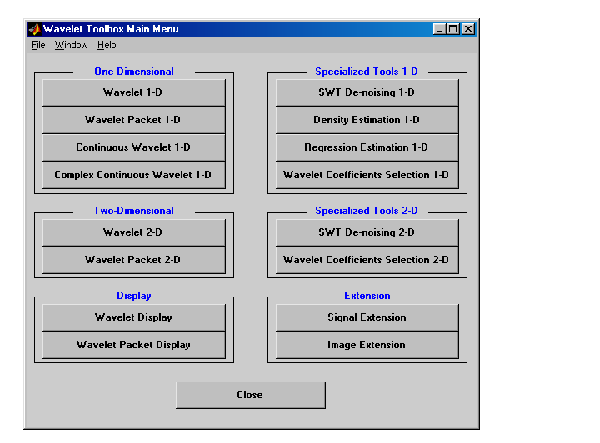Wavelet ToolboxStart graphical user interface tools

Syntax

• ```wavemenu
```

Description

`wavemenu` brings up a menu for accessing the various graphical tools provided in the Wavelet Toolbox. For instructions on using these tools see the corresponding chapters in the "MATLAB Wavelet Toolbox" User's Guide.

 Tools Chapter Wavelet 1-D and Wavelet 2-D Chapter 2 `Wavelet Packet 1-D and Wavelet Packet 2-D` Chapter 5 `Continuous Wavelet 1-D ` Chapter 2 `Complex Continuous Wavelet 1-D` Chapter 2 Wavelet Display and Wavelet Packet Display Chapter 1 `SWT De-noising 1-D and SWT De-noising 2-D` Chapter 2 `Density Estimation1-D` Chapter 2 `Regression Estimation1-D` Chapter 2 `Wavelet Coefficients Selection 1-D ` Chapter 2 `Wavelet Coefficients Selection 2-D ` Chapter 2 `Signal Extension and Image Extension` Chapter 2

The title of each mentioned chapter is given in the following table.

 Chapter Title Chapter 1 "Wavelets: A New Tool for Signal Analysis" `Chapter 2` "Using Wavelets" `Chapter 5` "Using Wavelet Packets"

Examples

• ```wavemenu```waveinfo wavemngr